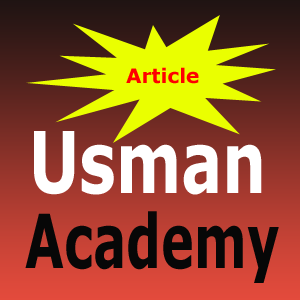# C/ C++ Programming MCQ's Question with Solution Multiple Choice Question Online Part 1

Part 1 C/ C++ Programming  MCQs Question with Solution Quiz Question Multiple Choice Question Online

1. What is wrong with the following: int long = 345000?
a. Invalid data type
b. Out of range value
c. Invalid name for variable
d. All of the above

2. Every statement in C++ program should end with
a. full stop (.)
b. Comma (,)
c. Semicolon (;)
d. colon (:)

3. Which of the following is not a correct variable type?
a. float
b. real
c. int
d. double

4. Char Variable data type use how many storage.
a. 8 Bytes
b. 4 Bytes
c.1 Bytes
d. Non of the Above

5. What is the only function all C++ programs must contain?
a. start ()
b. system()
c. main()
d. program()

6. What will be print after executing following statement. (ASCII value of ‘A’ is 65)
int value=65;
char ch=value;
cout<<ch;

a. ‘A’
b. 65
c. Error
d. Non of the Above

7. What does the following evaluate to: 12*6/4+592/3*49?
a. 1225
b. 77
c. 0.5374
d. 0.008

SOLUTION

1. What is wrong with the following: int long = 345000?
a. Invalid data type
b. Out of range value
c. Invalid name for variable
d. All of the above

2. Every statement in C++ program should end with
a. full stop (.)
b. Comma (,)
c. Semicolon (;)
d. colon (:)

3. Which of the following is not a correct variable type?
a. float
b. real
c. int
d. double

4. Char Variable data type use how many storage.
a. 8 Bytes
b. 4 Bytes
c.1 Bytes
d. Non of the Above

5. What is the only function all C++ programs must contain?
a. start ()
b. system()
c. main()
d. program()

6. What will be print after executing following statement. (ASCII value of ‘A’ is 65)
int value=65;
char ch=value;
cout<<ch;

a. ‘A’
b. 65
c. Error
d. Non of the Above

7. What does the following evaluate to: 12*6/4+592/3*49?
a. 1225
b. 77
c. 0.5374
d. 0.008• Title : C/ C++ Programming MCQ's Question with Solution Multiple Choice Question Online Part 1
• Posted by :
• Date : 11:56
• Labels :

1.2.